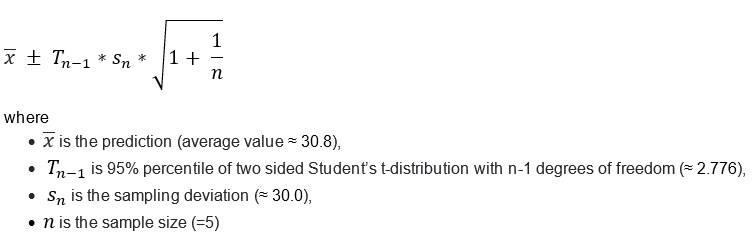Q: The read-across prediction is equal to 30.8 (the mean value of: 72.1, 50.0, 25.0, 3.787, 3.230). With a 95% level of significance (default value) the left and right endpoints of this prediction are computed as being -60.4 and 122. Given that the standard deviation (unbiased estimation, use of “n-1” at the denominator) of the five EC3 values associated with the five closest neighbors is equal to 30, I expected the endpoints to be equal to:

Left endpoint (95% level) = 30.8 - 1.96*30 =  -28

Right endpoint (95% level) = 30.8 + 1.96*30 =  89.6

On the other hand, it would seem that values approaching a 99.7% interval are computed instead:

Left endpoint (99.7% level) = 30.8 - 3*30 =  -59.2

Right endpoint (99.7% level) = 30.8 + 3*30 =  120.8

Do you have a clearer understanding about the computation of prediction confidence range?

A: The confidence interval for predictions based on samples coming from distributions with unknown mean and unknown variance is:After calculating you will see that:

EC3min ≈ -60.4

EC3max ≈ 122.0

The term Tn-1 takes into account the sample size and is important for small samples.

If sample size goes to infinity Tn-1 ≈ 1.96 as it is in your calculations.

(This question is also posted at the Toolbox Discussion forum: https://community.oecd.org/thread/27296 )